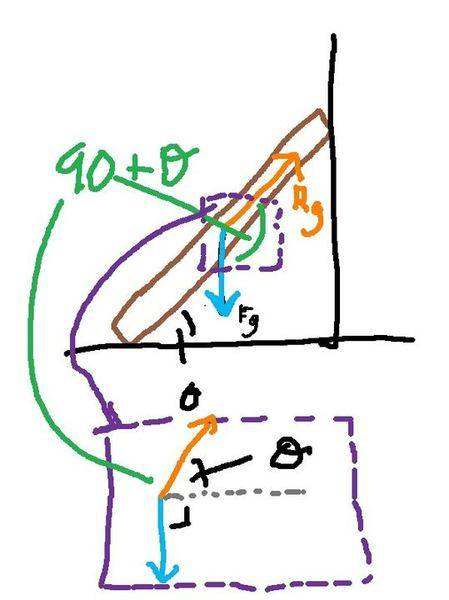# Static equilibrium, torque

• lemonpie
In summary, a 75 kg window cleaner using a 10 kg ladder that is 5.0 m long placed one end on the ground 2.5 m from a wall and climbed up 3m before the window broke. Neglecting friction and assuming the base of the ladder did not slip, the magnitude of the force on the window from the ladder was 282.9 N. The magnitude of the force on the ladder from the ground was 122.5 N and the angle of that force on the ladder relative to the horizontal was 60 degrees. The main difficulty in solving this problem was understanding the direction of the forces and the use of r in calculating torque.

## Homework Statement

A 75 kg window cleaner uses a 10 kg ladder that is 5.0 m long. He places one end on the
ground 2.5 m from a a wall, rests the upper end against a cracked window and climbs the ladder. He is 3m up along the ladder when the window breaks. Neglect friction between the ladder and window and assume that the base of the ladder does not slip. When the window is on the verge of breaking, what are (a) the magnitude of the force on the window from the ladder, (b) the magnitude of the force on the ladder from the ground and (c)the angle (relative to the horizontal of that force on the ladder.

## Homework Equations

net torque = r1F1 + r2F2 + r3F3

## The Attempt at a Solution

i have spent literally two hours on this one question, drawing triangles and diagrams over and over. i think my main problem is that i have no idea what to use for r. is it horizontal or vertical or along the angle of the ladder? every way i try, it doesn't come out to the correct answer. i also lack an understanding of what forces act in this situation.

F1 = weight of man on ladder
F2 = force of... window on ladder?
F3 = force of ladder on window

r1 = ?
r2 = ?
r3 = ?

net torque = (75*9.8)r1 + (10*9.8)r2 + F3r3 = 0

If you could show your diagrams for your working, that would help.

Without answering it for you, I'll give you some hints that might get you over some common mistakes:

1/ You can choose any point to find equilibrate your torques around. It is a good idea to choose a point where you do not know the magnitude of the force acting (hence at r = 0, rF = 0) and it eliminates that unknown from your equation.

2/ The forces you use to calculate your torques should be perpendicular (at 90 degrees) from the direction you use to calculate r.

3/ When calculating your torques, ONLY consider the forces acting on ONE OBJECT. Don't get yourself confused.

1. i chose the base of the ladder as the origin, i guess because i don't know that force.

2. so i think this is what i don't understand. my forces basically go straight downward, vertically. so the direction of r has to be... horizontal?

3. so i can only consider the forces acting on... the window? so my F2 is wrong?

okay, i think i found the right answer, but I'm not really sure why it's right.

net torque = (75)(9.8)3sin30 + (10)(9.8)2.5sin30 - 4.33F3 = 0
1102.5 + 122.5 - 4.33F3 = 0
F3 = 282.9 N

the part i don't understand is (4.33)(F3). why does r = 4.33? that would mean that r just goes straight down and the force must be horizontal. the other two r's are horizontal, and the force goes straight down.

lemonpie said:
1. i chose the base of the ladder as the origin, i guess because i don't know that force.

2. so i think this is what i don't understand. my forces basically go straight downward, vertically. so the direction of r has to be... horizontal?

3. so i can only consider the forces acting on... the window? so my F2 is wrong?

For number 2, I would keep your forces point down, and I would put R along the ladder (body).

What would help is to put R and F tail to tail, so you can see the angle between them. So with Fg (halfway up he ladder) pointing straight down, start the Rg vector at the tail of Fg, pointing up along the ladder toward the window.. The angle to use when calculating torque would be (90+theta)

So R*sin(90+theta)*Fg.. Same angle for all forces pointing straight down.

If you are also working with F normal from the window on the ladder, that would be sin(180-theta)..

Like this:
*note- this is only 1 force (gravity).. R is the distance from point of rotation (base of ladder) to Fg..
It is color coded to try and help separate what I'm sayingLast edited: